Next: 2-d problem with Neumann Up: The diffusion equation Previous: An improved 1-d solution

## 2-d problem with Dirichlet boundary conditions

Let us consider the solution of the diffusion equation in two dimensions. Suppose that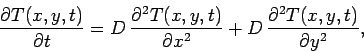(214)

for, and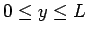. Suppose that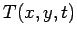satisfies mixed boundary conditions in the-direction: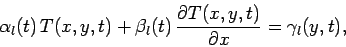(215)

at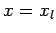, and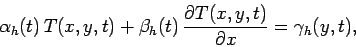(216)

at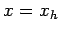. Here,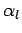,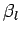, etc., are known functions of, whereas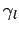,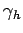are known functions ofand. Furthermore, suppose thatsatisfies the following simple Dirichlet boundary conditions in the-direction: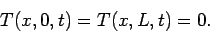(217)

As before, we discretize in time on the uniform grid, for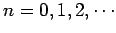. Furthermore, in the-direction, we discretize on the uniform grid, for, where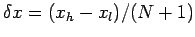. Finally, in the-direction, we discretize on the uniform grid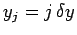, for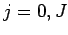, where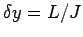. Adopting the Crank-Nicholson temporal differencing scheme discussed in Sect. 6.6, and the second-order spatial differencing scheme outlined in Sect. 5.2, Eq. (214) yields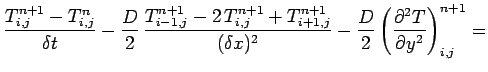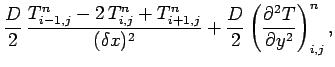(218)

where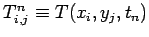. The discretized boundary conditions take the form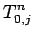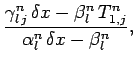(219)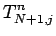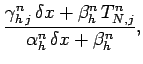(220)

plus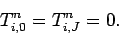(221)

Here,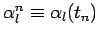, etc., and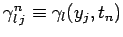, etc.

Adopting the Fourier method introduced in Sect. 5.7, we write the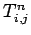in terms of their Fourier-sine harmonics: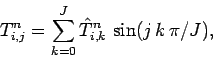(222)

which automatically satisfies the boundary conditions (221). The above expression can be inverted to give (see Sect. 5.9)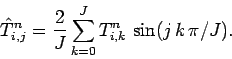(223)

When Eq. (218) is written in terms of the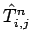, it reduces to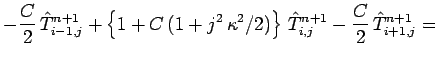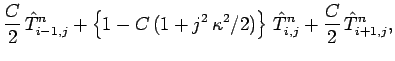(224)

for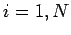, and. Here,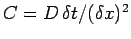, and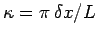. Moreover, the boundary conditions (219) and (220) yield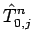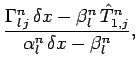(225)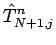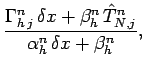(226)

where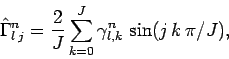(227)

etc. Equations (224)--(226) constitute a set of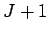uncoupled tridiagonal matrix equations for the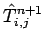, with one equation for each separate value of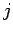.

In order to advance our solution by one time-step, we first Fourier transform theand the boundary conditions, according to Eqs. (223) and (227). Next, we invert thetridiagonal equations (224)--(226) to obtain the. Finally, we reconstruct thevia Eq. (222).Next: 2-d problem with Neumann Up: The diffusion equation Previous: An improved 1-d solution
Richard Fitzpatrick 2006-03-29